# Summary of Current Mode Transfer Functions and Various Modes

2017.03.09

・Understand the differences in the slope waveforms and duty cycle control for voltage mode and current mode.

・When deriving a transfer function, be sure to adequately comprehend the previous sections of "Voltage-Mode Transfer Functions" and "Consideration of Current Mode ".

As a slope transfer function, “Voltage-Mode Transfer Functions” were derived, and then “Consideration of Current Mode” was conducted. This time, we will derive the current mode transfer functions, while comparing and contrasting with the voltage mode, and will summarize the voltage-mode and current-mode transfer functions.

## Comparison of voltage mode and current mode

Before deriving the current mode transfer functions, we compare the voltage mode and current mode, including general approaches to these concepts.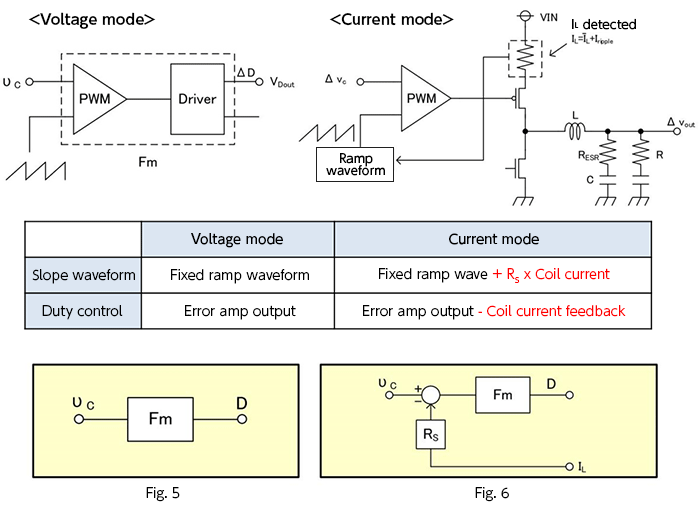Starting from the top, these are schematic circuit diagrams, a table indicating the differences in slope waveform and duty control for the two modes, and, in Fig. 5 and Fig. 6, block diagrams of the voltage mode and current mode based on the table.

## Current mode transfer function

From here, we begin to consider current mode transfer functions. To begin with, in the current mode block diagram of Fig. 6 above, using the duty cycle and taking Gdi to be the transfer function for the inductor current IL, equation 3-5 is obtained.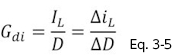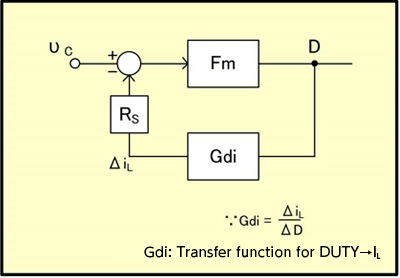Fig.7

Using this Gdi to modify Fig. 6, we can obtain the representation of Fig. 7. As indicated by equation 3-5, Gdi can be represented as ΔiL divided by ΔD.
Further, based on Fig. 7, an equation for D can be written using Vc, to obtain equation 3-6 below.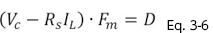Further, upon substituting a perturbation into equation 3-6 and using equation 3-5 to determine the transfer function, we obtain equation 3-7.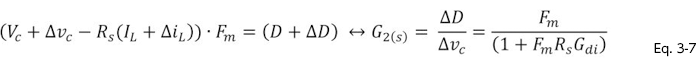Fig. 8 shows the effect on the slope waveform of the perturbation of equation 3-7.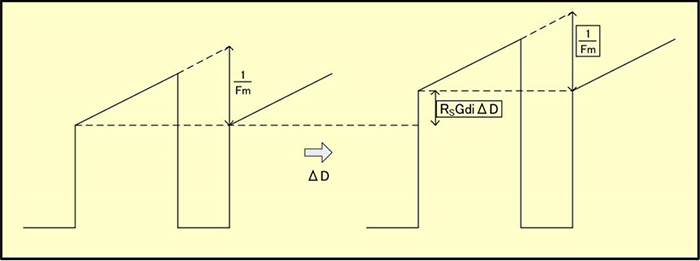Fig.8

Here,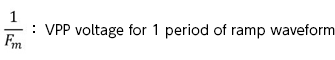Further, we can say that in current mode, it is desirable that a design be used that satisfies the condition of equation 3-8 below.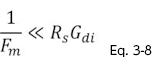By satisfying this condition, current mode can alleviate the effect of the coil inductance L, so that first-order system characteristics are exhibited.
As a final summary, we compare the transfer functions and block diagrams of voltage mode and current mode.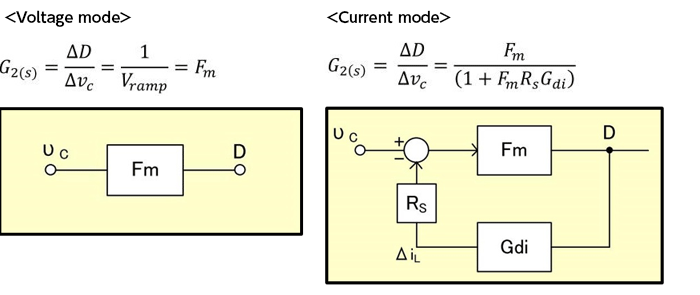Next time, we will derive the Fm transfer function in current mode.